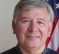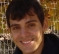## How does one delay a signal and allow its phase to propagate vs time with a specific slope or rate?Started by 6 years ago10 replieslatest reply 6 years ago121 views
How does one delay a signal and allow its phase to propagate vs time with a specific slope or rate? How can one -digitally- simulate the effect of a propagation line, in the sense that, depending on the line's lenght, the output signal's phase will change? A FIR filter for e.g. has a linear phase response vs frequency, and a signal output from such a filter will be delayed in time, but will maintain its original phase charecteristics, and not allow the phase to change depending on its group-delay length. Is there another filter that can do this? Thank you
[ - ]Hi,

A delay of length T can be implemented by a series of one-sample delays, and it will have a linear phase slope.  For example, if you want a delay of 5 ms and your have a sample time of 1 ms, you would use 5 delay elements.  Each delay element is z^-1.  This is the equivalent of a "propagation line", by which I assume you mean a length of transmission line.  It is not necessary to implement as a FIR filter, although a symmetric FIR filter does have linear phase.

The Laplace transform of delay T is:  u(t - T) --> e^-sT.  The z-transform of delay Ts is simply z^-1.

regards,

Neil

[ - ]Hi Neil, thank you very much for your reply. I will try and simulate a delay line as you suggested. I have realized that perhaps I could make the question a lot more clear by a diagram. I have done so in a new post. Your input would be much appreciated.

[ - ]A few fundamentals:

"phase" is a relative term that is tied to "time=0".  Sorry if that's an obvious assertion but it may come up next...

Any time delay causes a phase shift (releative to? .. see above) that is frequency-dependent.  So, zero delay is  zero phase shift, etc.  It should be obvious why it's a function of frequency.  And, it should be easy to see that a given delay will give a phase shift value that's linear in FREQUENCY.  That's the normal sort of thing that is referred to with linear phase FIR filters.

I'm not sure what you mean by:

"How does one delay a signal and allow its phase to propagate vs time with a specific slope or rate?"

1) Do you mean: "How does one delay a sinusoid and have its phase change vs. time with a linear slope or rate?"

2) Do you mean: "How does one delay a sinusoid and have its phase change vs. frequency with a linear slope or rate?"

3) Do you mean: "How does one delay a general signal and have its phase change vs. anything with a linear slope or rate?"

1) You have to provide a linearly-varying time delay which might be implemented with a memory and a time-varying address to retrieve the data.  Of course, for this to work very long would take a lot of memory OR a time-varying window into the memory in use.

2) You have to provide a single time delay.  That's all.

3) The question doesn't make sense because "phase" only applies to a single frequency at a time.  But maybe you can use the answer to (1) to get a time-varying delay on a general signal which will then have time-varying phase AT EACH frequency - with the rates being dependent on frequency.

This is mostly just physics.  I hope it's helpful.

[ - ]Hi Fred, thank you very much for your reply. I appreciate you taking it bottom-up, since I am a SW man with not much signal processing under my belt.
I indeed meant number (1). I will try and simulate a memory as you suggested. I have realized that perhaps I could make the question a lot more clear by a diagram. I have done so in a new post. Your input would be much appreciated.

[ - ]IIR has nonlinear delay if that is what you are after

[ - ]Thank you for your reply. I have tried to clarify the question in a separate new post.

Your ideas would be much appreciated.

[ - ]Multiply the delayed samples by a phase ramp.

See the properties of the Fourier transform.

[ - ]Thank you for your reply. I have tried to clarify the question in a separate new post.

I am unable to use Fourier - as you will see in my new post -because the signal has a limited observation time = limited number of samples = worst resolution for the frequency axis w. (frequency resolution = 1/observation_time).

[ - ]